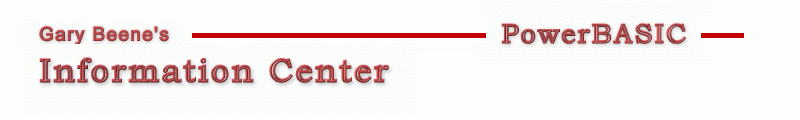## Sprite 01 - RealTime, No Buffer, 1 Sprite

Category: Sprite Tutor Series

Date: 03-28-2012

'Compilable Example:
#Compiler PBWin 9, PBWin 10
#Compile EXE
#Dim All
#Include "Win32API.inc"
#Resource "gbsnippets.pbr"

Type BallInfo
w As Long         'width (of enclosing rectangle)
h As Long         'height (of enclosing rectangle)
x As Long         'x-position (upper-left corner)
y As Long         'y-position (upper-left corner)
sx As Long        'distance to move in x direction (speed)
sy As Long        'distance to move in y direction (speed)
framecur As Long  'current Frame #, the one to be displayed
framemax As Long  'total number of available frames For the sprite
waitcnt As Long   'current wait count (counts Timer Events)
waitmax As Long   'max wait count before changing frames
hnd as DWord      'image handle or arra index of sprite
End Type

Global hDlg As DWord, ball As BallInfo, iCount as Long
Global canvasW as Long, canvasH as Long
%ID_Graphic = 300 : %ID_Timer = 400 : %Delta = 2

Function PBMain() As Long
canvasW = 300 : canvasH = 300
Dialog New Pixels, 0, "Graphic Control Test",300,300,400,400, %WS_SysMenu, 0 To hDlg
Control Add Graphic, hDlg, %ID_Graphic,"", 0,0,canvasW,canvasH, %WS_Visible Or %SS_Sunken
Graphic Attach hDlg, %ID_Graphic
Graphic Color %Black,%White
Graphic Clear
Dialog Show Modal hDlg Call DlgProc
End Function

CallBack Function DlgProc() As Long
Select Case CB.Msg
Case %WM_InitDialog
DefineBall
SetTimer(CB.Hndl, %ID_Timer, 20, ByVal %NULL)   'uses callback messages
Case %WM_Timer
Incr iCount : Dialog Set Text hDlg, Str\$(iCount)
MoveBall
DrawBall
Control Redraw hDlg, %ID_Graphic
Case %WM_Destroy
KillTimer CB.Hndl, %ID_Timer
Case %WM_Command
If CB.Ctl = %ID_Graphic Then

End If
End Select
End Function

Sub DrawBall
Graphic Clear
Graphic Ellipse (ball.x, ball.y)-(ball.x + ball.w, ball.y + ball.h), %Black, %Blue
End Sub

Sub MoveBall
ball.x = ball.x + ball.sx
ball.y = ball.y + ball.sy

If ball.x < 0 Then
ball.x = 0
ball.sx = %Delta
ElseIf ball.x + ball.w > canvasW Then
ball.x = canvasW - ball.w
ball.sx = - %Delta
End If

If ball.y < 0 Then
ball.y = 0
ball.sy = %Delta
ElseIf ball.y + ball.h > canvasH Then
ball.y = canvasH - ball.h
ball.sy = - %Delta
End If
End Sub

Sub DefineBall
Ball.w = 30
Ball.h = 30
Ball.x = 50
Ball.y = 150
Ball.sx = %Delta
Ball.sy = %Delta
Ball.framecur = 0    'zero based frame count
Ball.framemax = 0    'only 1 frame in this example
Ball.waitcnt = 1     'one based - current wait count (counts Timer Events)
Ball.waitmax = 1     'one based - # timer events before changing frames
Ball.hnd = 0         'image handle or arra index of sprite
End Sub

'void DrawBall(HDC hdc, RECT* prc)
'{
'    HDC hdcBuffer = CreateCompatibleDC(hdc);
'    HBITMAP hbmBuffer = CreateCompatibleBitmap(hdc, prc->right, prc->bottom);
'    HBITMAP hbmOldBuffer = SelectObject(hdcBuffer, hbmBuffer);
'
'    HDC hdcMem = CreateCompatibleDC(hdc);
'    HBITMAP hbmOld = SelectObject(hdcMem, g_hbmMask);
'
'    FillRect(hdcBuffer, prc, GetStockObject(WHITE_BRUSH));
'
'    BitBlt(hdcBuffer, g_ballInfo.x, g_ballInfo.y, g_ballInfo.Width, g_ballInfo.height, hdcMem, 0, 0, SRCAND);
'
'    SelectObject(hdcMem, g_hbmBall);
'    BitBlt(hdcBuffer, g_ballInfo.x, g_ballInfo.y, g_ballInfo.Width, g_ballInfo.height, hdcMem, 0, 0, SRCPAINT);
'
'    BitBlt(hdc, 0, 0, prc->right, prc->bottom, hdcBuffer, 0, 0, SRCCOPY);
'
'    SelectObject(hdcMem, hbmOld);
'    DeleteDC(hdcMem);
'
'    SelectObject(hdcBuffer, hbmOldBuffer);
'    DeleteDC(hdcBuffer);
'    DeleteObject(hbmBuffer);
'
'

'gbs_00450
'Date: 03-10-2012

created by gbSnippets
http://www.garybeene.com/sw/gbsnippets.htm